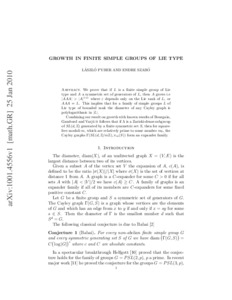REAL

# Growth in finite simple groups of Lie type

Pyber, László and Szabó, Endre (2016) Growth in finite simple groups of Lie type. JOURNAL OF THE AMERICAN MATHEMATICAL SOCIETY, 29. pp. 95-146. ISSN 0894-0347Text
growth.JAMS.pdf - Published Version
Restricted to Registered users onlyPreview
Text
44517.pdf - Submitted Version

We prove that if $L$ is a finite simple group of Lie type and $A$ a set of generators of $L$, then either $A$ grows, i.e., $\vert A^3\vert > \vert A\vert^{1+\varepsilon }$ where $\varepsilon$ depends only on the Lie rank of $L$, or $A^3=L$. This implies that for simple groups of Lie type of bounded rank a well-known conjecture of Babai holds, i.e., the diameter of any Cayley graph is polylogarithmic. We also obtain new families of expanders. A generalization of our proof yields the following. Let $A$ be a finite subset of $SL(n,\mathbb{F})$, $\mathbb{F}$ an arbitrary field, satisfying $\big \vert A^3\big \vert\le \mathcal {K}\vert A\vert$. Then $A$ can be covered by $\mathcal {K}^m$, i.e., polynomially many, cosets of a virtually soluble subgroup of $SL(n,\mathbb{F})$ which is normalized by $A$, where $m$ depends on $n$. - See more at: http://www.ams.org/journals/jams/0000-000-00/S0894-0347-2014-00821-3/home.html#sthash.Lp65MZge.dpufEdit Item